# Electromagnetic Induction And Alternating Current Class 12 Pdf Do

Chapter 4 ELECTROMAGNETIC INDUCTION AND ALTERNATING CURRENTS

## ELECTROMAGNETIC INDUCTION AND ALTERNATING CURRENTS

• The phenomenon of production of induced emf in a conductor when electric flux linked with that changes is called electromagnetic induction.

• Magnetic flux through a surface of area A placed in a uniform magnetic field B is defined as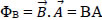Cosθ where θ is the angle between B and A.

• Magnetic flux is a scalar quantity and its SI unit is weber (Wb).

#### • Faraday’s laws of induction:

First Law: When magnetic flux linked with the conductor changes, induced emf produces across it. Second Law:

The magnitude of the induced e.m.f in a circuit is equal to the rate of change of magnitude flux linked with that circuit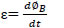#### • Lenz law:

The direction of induced current or the polarity of the induced e.m.f is in such a way that it opposes the cause that produces it. (The negative sign in Faraday’s law indicates this fact.)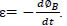Lenz law obeys the principle of energy conservation.

• The induced current in a closed loop can be produced by changing the
(i) magnitude of B
(ii) area A of the loop
(iii) its orientation in magnetic field.

• The direction of induced current is obtained from Fleming’s right hand rule.

• When a metal rod of length l is placed normal to a uniform magnetic field B and moved with a velocity v perpendicular to the field, the induced e.m.f is called motional e.m.f. It produces across the ends of the rod. ε = Blv.If ‘R’ is the resistance of the metal rod, the induced current is given by I=Blv/R, the force acting on the conductor in the presence of magnetic field (due to drift of charge) is given by F =B2l2v / R. The power required to move the conductor with a constant speed is given by P= B2l2v2/R.

• The induced currents produced on the surface of a thick conductor when magnetic flux linked with that changes are called eddy currents.

• The phenomenon of production of induced emf in a coil itself when electric current passing through that changes is called self induction. Self Inductance is the ratio of the flux linkage to current. =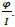• When a current in a coil changes it induces a back e.m.f in the same coil. The self induced e.m.f is given by ε =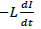where L is the self-inductance of the coil. It is a measure of inertia of the coil against the change of current through it. Its S.I unit is henry (H).

• The inductance is said to be one Henry when an emf of one volt induces in a coil for unit rate of change of electric current through it.

• The changing current in a coil can induce an e.m.f in a nearby coil. This relation, ε =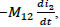shows that Mutual inductance of coil 1 with respect to coil 2 (M12) is due to change of current in coil 2. (M12 =M21).

• The self-inductance of a long solenoid is given by L = μ0n2Al where A is the area of cross-section of the solenoid, l is its length and n is the number of turns per unit length.

• The mutual inductance of two co-axial coils is given by M12 = M21 = μM0 n1n2Al where n1& n2 are the number of turns per unit length of coils 1 & 2. A is the area of cross-section and l is the length of the solenoids.

• Energy stored in an inductor in the form of magnetic field is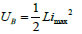and Magnetic energy density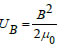• The electric current whose magnitude changes continuously and direction changes periodically is called alternating current (AC). I = Io Sin ωt. • The root mean square value of a.c. may be defined as that value of steady current which would generate the same amount of heat in a given resistance in a given time as is done by the a.c. when passed through the same resistance during the same time. Irms = Io/√2 = 0.707i0 . Similarly, vrms = vo/√2 = 0.707vo

• The rotating vectors which represent the varying quantities are called phasors. The diagram in which the AC voltage and AC currents are represented as phasors is called phasor diagram.

• The opposition offered by resistor is called resistance (R). The non-resistive opposition offered by a device is called reactance (X). The combination of reactance and resistance is called impedance (Z).

• An alternating voltage ε=ε0Sinωt, applied to a resistor R drives a current I = I0Sinωt in the resistor, I0 = ε0 /R where ε0& I0 are the peak values of voltage and current. (also represented by Vm & Im)

• For an AC emf ε = εm Sin ωt applied to a resistor, current and voltage are in phase.

• In case of an a.c. circuit having pure inductance current lags behind e.m.f by a phase angle 90°. ε = εm Sin ωt and i = im Sin (ωt-π/2). Im = εm/XL; XL = ωL is called inductive reactance.

• In case of an a.c. circuit having pure capacitance, current leads e.m.f by a phase angle of 90°. ε = εmSinωt and I= ImSin(ωt+π/2) where Im = εm/XC and XC = 1/ωC is called capacitive reactance.

• In case of an a.c. circuit having R, L and C, the total or effective resistance of the circuit is called impedance (Z).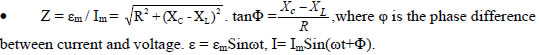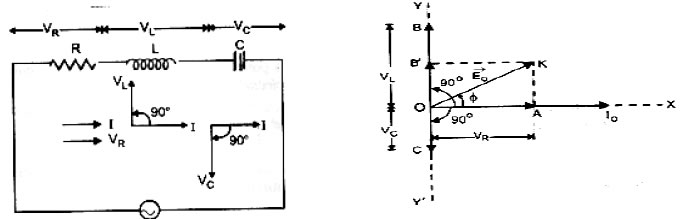• Average power loss over a complete cycle in an LCR circuit is P = εrmsIrmsCosΦ

• In a purely resistive circuit Φ = 0; P = VRMSIRMS.
• In a purely inductive circuit Φ = π/2; P = 0.
• In a purely capacitive circuit Φ = π/2; P = 0.

• The electric current in an AC circuit is said to be wattless current when average power dissipated or consumed is zero.

• In an LCR circuit, the circuit admits maximum current if XC = XL, so that Z = R and resonant frequency wr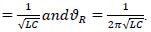• The device which converts mechanical energy in to AC electrical energy by virtue of electromagnetic induction is called AC Generator.

• Rotation of rectangular coil in a magnetic field causes change in flux (Φ = NBACosωt). Change in flux induces e.m.f in the coil which is given by ε= -dΦ/dt = NBAωSinωt. ε = ε0Sinωt. Current induced in the coil I = ε/R = ε0Sinωt/R = I0Sinωt

• The device which converts an AC voltage of one value to another is called Transformer. For an ideal transformer,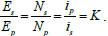• In an ideal transformer, εPIP = εSIS. i.e

• If NS>NP; εS>εP& IS< IP – step up. If NP>NS; εP>εS & IPIS – step down.

• Losses in transformer: Copper losses; Iron losses, Flux losses; Hysteresis losses; Humming losses.

• When a charged capacitor is allowed to discharge through an inductor, electrical oscillations of constant amplitude and frequency are produced, which is called LC oscillations. The charge on capacitor q satisfies the equation of SHM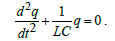### NCERT Books Free Pdf Download for Class 5, 6, 7, 8, 9, 10 , 11, 12 Hindi and English Medium

 Mathematics Biology Psychology Chemistry English Economics Sociology Hindi Business Studies Geography Science Political Science Statistics Physics Accountancy